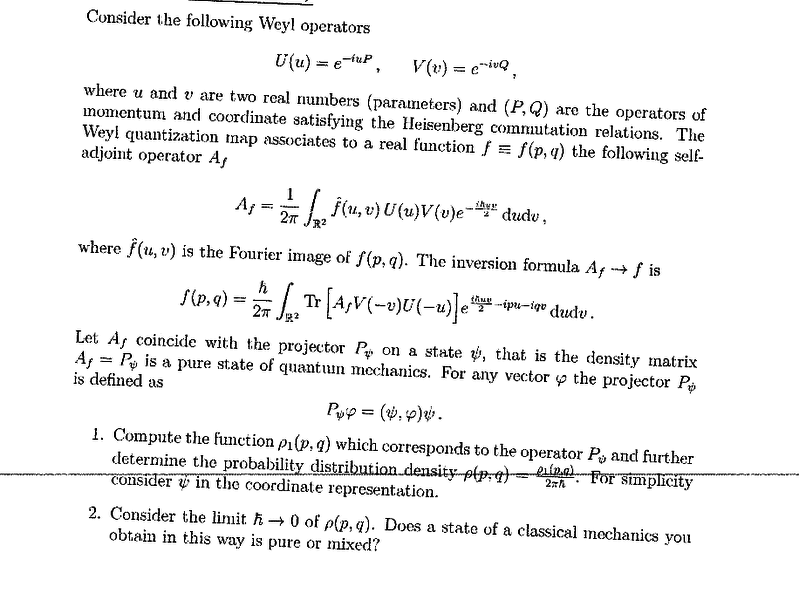# Inverse Weyl quantization of the projection operator.

I am trying to solve the following problem on an old Quantum Mechanics exam as an exercise.

## Homework Statement## Homework Equations

I know that the trace of an operator is the integral of its kernel.
\begin{equation}
Tr[K(x,y)] = \int K(x,x) dx
\end{equation}

## The Attempt at a Solution

I start out by finding the action of the operator in the trace:
\begin{equation}
(P_{\psi} V(-v) U(-u) \phi)(x) = \int \psi^{\star} e^{i v x} \phi(x+\hbar u) dx \psi(x)
\end{equation}
Which I think should, by sending $x\rightarrow y-\hbar u$, be equal to:
\begin{equation}
\int \psi^{\star}(y- \hbar u) e^{i v (y-\hbar u)} \phi(y) \psi(x) dy
\end{equation}
Which means that the integral kernel of this operator is:
\begin{equation}
K_{\psi}(x,y) = \psi^{\star}(y- \hbar u) e^{i v (y-\hbar u)} \psi(x)
\end{equation}
Which means that the trace is:
\begin{equation}
\int K_{\psi}(x,x) dx = \int \psi^{\star}(x- \hbar u) e^{i v (x-\hbar u)} \psi(x) dx
\end{equation}

So essentially I am stuck trying to solve this integral.
Any help would be greatly appreciated.

#### Attachments

TSny
Homework Helper
Gold Member
Which means that the trace is:
\begin{equation}
\int K_{\psi}(x,x) dx = \int \psi^{\star}(x- \hbar u) e^{i v (x-\hbar u)} \psi(x) dx
\end{equation}

So essentially I am stuck trying to solve this integral.
Any help would be greatly appreciated.

Use this expression for the trace in the equation for ##f(q,p)## and see if you can do the ##v## integration next and then do the ##x## integration.

Thanks!
I gave that a try, and this is what I came up with:

\begin{equation}
f(p,q) = \frac{\hbar}{2 \pi} \int_{ℝ^{2}} \int_{ℝ} \psi^{\star}(x-\hbar u) e^{ev(x-\hbar u)} \psi(x) e^{i \hbar u v/2 -ipu -iqv/} du dv dx
\end{equation}

Which I think should be combined as follows so I get an expression for the dirac-delta distribution:

\begin{equation}
\frac{\hbar}{2 \pi} \int_{ℝ^{2}} \int_{ℝ} \psi^{\star}(x - \hbar u) \psi(x) e^{-ipu} e^{iv(-\hbar u/2 + x -q)} du dv dx
\end{equation}

Where the v integration gives the delta distribution:
\begin{equation}
f(p,q) = \frac{\hbar}{\sqrt{2 \pi}} \int_{ℝ} \int_{ℝ} \psi^{\star}(x-\hbar u) \psi(x) e^{-ipu} \delta(\frac{-\hbar u}{2} + x -q) dudx
\end{equation}
I figure you could do either the x or the u integration here, but since you said I should start with the x integration:
\begin{equation}
f(p,q) = \frac{\hbar}{\sqrt{2 \pi}} \int_{ℝ} \psi^{\star}(\frac{-\hbar u}{2} + q) \psi(\frac{\hbar u}{2} + q) e^{-ipu} du
\end{equation}

I don't think the last integration can be taken any further without knowing what psi is...

As for part b, in the limit that hbar approaches zero, does this function not becomes simply identically zero?

TSny
Homework Helper
Gold Member
Yes, you could do the u integration before the x integration. I think you should get the same result.

I don't think the factor of ##1/\sqrt{2\pi}## should be in the result. (Check the numerical factors in getting the delta function from the integration of the exponential.)

Note that the probability distribution is defined as ##f(p,q)/(2\pi\hbar)##, so the ##\hbar## in front of the integral in ##f(p,q)## will disappear. You can then try to get the classical limit.

Thanks again.
I believe the current prefactor $\frac{1}{\sqrt{2 \pi}}$ is still correct, but it will be taken care of once you divide by $2 \pi \hbar$ and compute the last integral, which is the fourier transform of $|\psi|^{2}$.

TSny
Homework Helper
Gold Member
Thanks again.
I believe the current prefactor $\frac{1}{\sqrt{2 \pi}}$ is still correct, but it will be taken care of once you divide by $2 \pi \hbar$ and compute the last integral, which is the fourier transform of $|\psi|^{2}$.

Maybe I'm overlooking a factor of ##\sqrt{2\pi}## somewhere. When integrating over ##v## to get the dirac delta function, did you use the integral representation of the delta function as given in equation 1.17.12 here ?

The last integral is not quite the Fourier transform of ##|\psi^2|## due to the difference in signs occurring inside the arguments of ##\psi## and ##\psi^*##.

I meant it would be the fourier transform in the classical limit. And you're right about me getting that factor wrong. I misremembered there being a square root.

TSny
Homework Helper
Gold Member
I meant it would be the fourier transform in the classical limit.

I'm not sure about the classical limit. It seems to me that if you let h $\rightarrow$ 0, then the arguments of ψ and ψ* no longer depend on the integration variable u. Thus you can pull ψ and ψ* out of the integral.